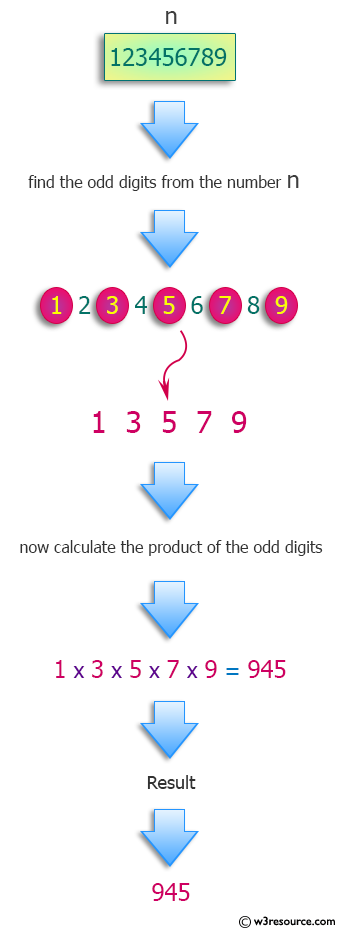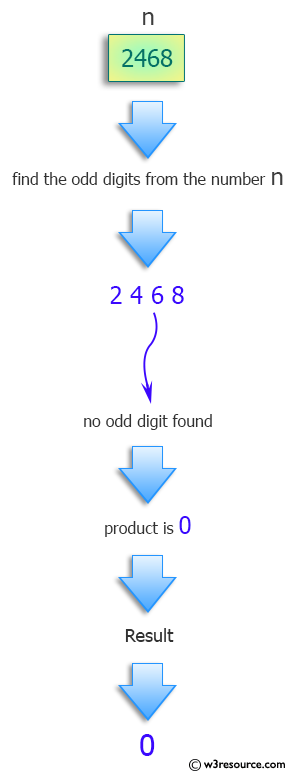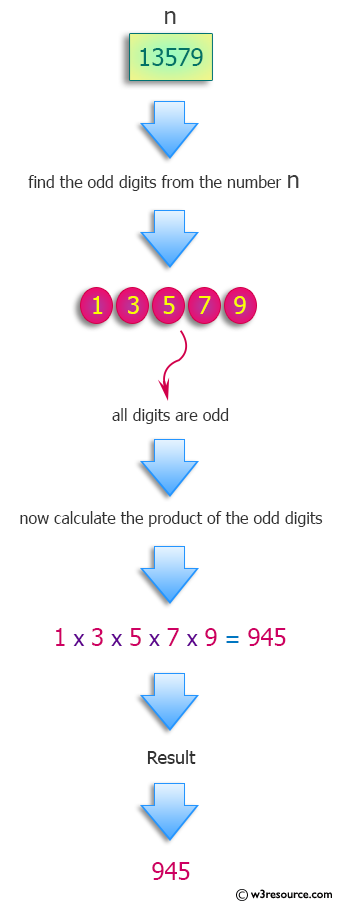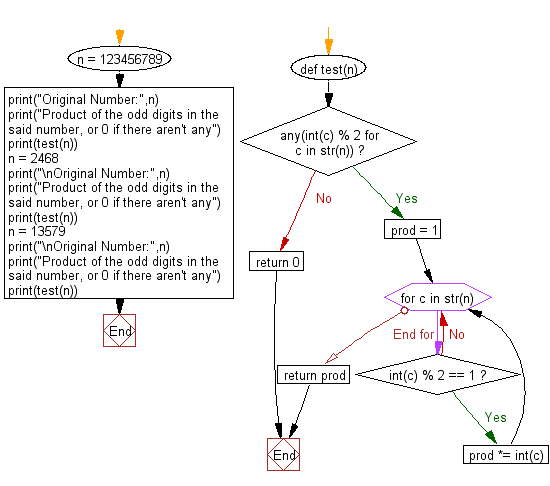﻿ Python: Product of the odd digits in n, or 0 if there aren't any - w3resource# Python: Product of the odd digits in n, or 0 if there aren't any

## Python Programming Puzzles: Exercise-35 with Solution

Write a Python program to compute the product of the odd digits in a given number, or 0 if there aren't any.

```Input:
123456789
Output:
945

Input:
2468
Output:
0

Input:
13579
Output:
945
```

Pictorial Presentation:Sample Solution:

Python Code:

``````#License: https://bit.ly/3oLErEI

def test(n):
if any(int(c) % 2 for c in str(n)):
prod = 1
for c in str(n):
if int(c) % 2 == 1:
prod *= int(c)
return prod
return 0

n = 123456789
print("Original Number:",n)
print("Product of the odd digits in the said number, or 0 if there aren't any")
print(test(n))
n = 2468
print("\nOriginal Number:",n)
print("Product of the odd digits in the said number, or 0 if there aren't any")
print(test(n))
n = 13579
print("\nOriginal Number:",n)
print("Product of the odd digits in the said number, or 0 if there aren't any")
print(test(n))
``````

Sample Output:

```Original Number: 123456789
Product of the odd digits in the said number, or 0 if there aren't any
945

Original Number: 2468
Product of the odd digits in the said number, or 0 if there aren't any
0

Original Number: 13579
Product of the odd digits in the said number, or 0 if there aren't any
945
```

Flowchart:## Visualize Python code execution:

The following tool visualize what the computer is doing step-by-step as it executes the said program:

Python Code Editor :

Have another way to solve this solution? Contribute your code (and comments) through Disqus.

What is the difficulty level of this exercise?

Test your Programming skills with w3resource's quiz.

﻿

## Python: Tips of the Day

Clamps num within the inclusive range specified by the boundary values x and y:

Example:

```def tips_clamp_num(num,x,y):
return max(min(num, max(x, y)), min(x, y))
print(tips_clamp_num(2, 4, 6))
print(tips_clamp_num(1, -1, -6))
```

Output:

```4
-1
```